# Two-dimensional NMR spectroscopy

## The 2D NMR - The idea of JEENER

The idea of Jeener consists in the stepwise increasing of the evolution time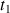.

This will allow to get an NMR signal under the aspect of a sampling of free precession signals of the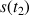type. These FID will differ from each other only by theperiod duration written under a matricial form

s(,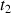). Thedelay is the time between the first and the second pulse.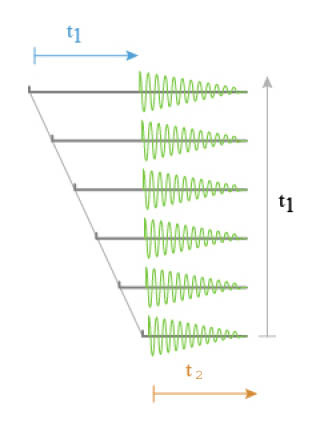Fig. 9 : Sampling of signals of free precession of the type s(t2)

The first Fourier transform as a function ofgives us an interferogram of the form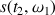(Fig. 10)Fig. 10 : Interferogram of the form s(t2, w1)

A second Fourier transform, versus the second variable, gives an NMR spectrum with two frequencies dimensions F1 and F2.

The result of this two fold Fourier transform does not get two spectra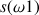and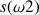but only one spectrum as a function of two independent frequencies, exhibiting a peak with the coordinates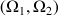. Thus, an aimantation evolving with the frequency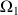in the time coursehas been converted in another coherence evolving with the frequencyduring the period.Fig. 11 : NMR spectrum in two dimensions following the second Fourier transform

This double Fourier transform in both dimension yields thus a matrix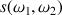(spectrum 3).